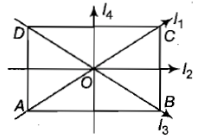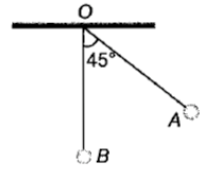The moment of inertia of a thin rectangular plate ABCD of uniform thickness about an axis passing through the centre O and perpendicular to the plane of the plate is1.

2.

3.

4.

Concept Questions :-

Moment of inertia
High Yielding Test Series + Question Bank - NEET 2020

Difficulty Level:

The centre of a wheel rolling on a plane surface moves with a speed ${\mathrm{v}}_{0}$. A particle on the rim of the wheel at the same level as the centre will be moving at speed

1.  zero

2.  ${\mathrm{v}}_{0}$

3.  $\sqrt{2}{\mathrm{v}}_{0}$

4.  $2{\mathrm{v}}_{0}$

Concept Questions :-

Rolling motion
High Yielding Test Series + Question Bank - NEET 2020

Difficulty Level:

A man weighing 80 kg is standing in a trolley weighing 320 kg. The trolley is resting on frictionless horizontal rails. If the man starts walking on the trolley with a speed of 1 m/s, then after 4 sec his displacement relative to the ground will be

(1) 5  m

(2) 4.8 m

(3) 3.2 m

(4) 3.0 m

Concept Questions :-

Center of mass
High Yielding Test Series + Question Bank - NEET 2020

Difficulty Level:

Moment of inertia of a uniform cylinder of mass M, radius R and length l about an axis passing through its center and normal to its axis would be

1.  $\frac{{\mathrm{Ml}}^{2}}{12}$

2.  $\frac{{\mathrm{MR}}^{2}}{2}$

3.

4.

Concept Questions :-

Moment of inertia
High Yielding Test Series + Question Bank - NEET 2020

Difficulty Level:

A particle moves along a circle of radius $\frac{20}{\mathrm{\pi }}m$ with constant tangential acceleration. If the velocity of the particle is 80 m/s at the end of the second revolution after motion has begin, the tangential acceleration is

(1) 640

(2) 160

(3) 40

(4) 40 $\mathrm{m}/{\mathrm{s}}^{2}$

Concept Questions :-

Rotation motion : Introduction
High Yielding Test Series + Question Bank - NEET 2020

Difficulty Level:

If the radius of the earth is suddenly contracted to half of its present value, then the duration of the day will be of

1.  6 hours

2.  12 hours

3.  18 hours

4.  24 hours

Concept Questions :-

Angular momentum
High Yielding Test Series + Question Bank - NEET 2020

Difficulty Level:

A wheel has angular acceleration of 3.0 rad/$se{c}^{2}$ and an initial angular speed of 2.00 rad/sec.  In a time of 2 sec it has rotated through an angle (in radian) of

(1) 10                (2) 12

(3) 4                  (4) 6

High Yielding Test Series + Question Bank - NEET 2020

Difficulty Level:

The bob A of a simple pendulum is released when the string makes an angle of 450 with the vertical. It hits another bob B of the same material and the same mass kept at rest on a table. If the collision is elastic1.  Both A and B rise to the same height

2.  Both A and B come to rest at B

3.  Both A and B move with the same velocity of A

4.  A comes to rest and B moves with the velocity of A

Concept Questions :-

Linear momentum
High Yielding Test Series + Question Bank - NEET 2020

Difficulty Level:

If a force acts on a body at a point away from the centre of mass, then

1.  Linear acceleration changes

2.  Angular acceleration changes

3.  Both change

4.  None of these

Concept Questions :-

Torque
High Yielding Test Series + Question Bank - NEET 2020

Difficulty Level:

If the linear density of a rod of length 3m varies as $\lambda$=2+x, then the position of the center of mass of the rod is at a distance of

1.  $\frac{7}{3}m$

2.  $\frac{10}{7}m$

3.  $\frac{12}{7}m$

4.  $\frac{9}{7}m$

Concept Questions :-

Center of mass